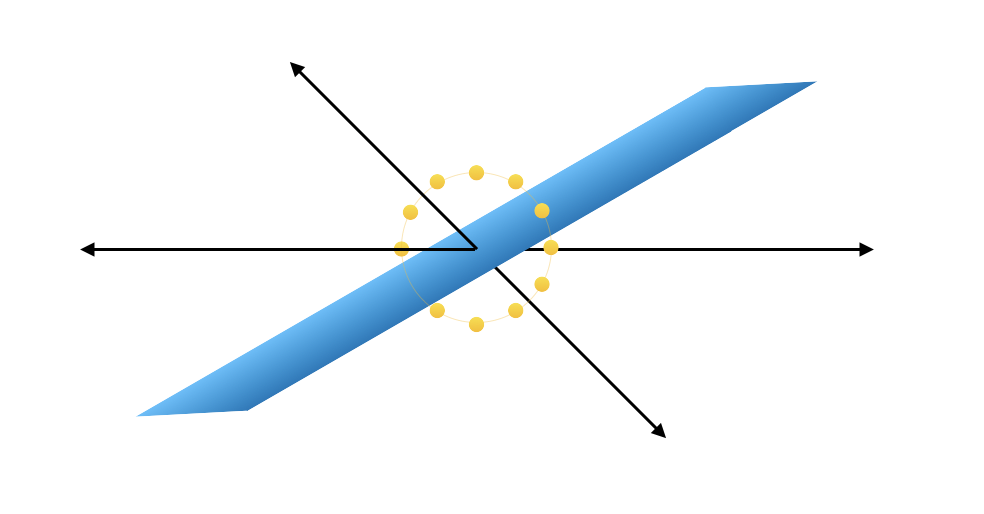# Estimating signal and noise in linear modelsLet $$\mathbf{Y} \in \mathbb{R}^n$$ and $$\boldsymbol{\beta} \in \mathbb{R}^p$$ and consider the model

\begin{aligned} \mathbf{Y} | \boldsymbol{\beta} &\sim N(\mathbf{X} \boldsymbol{\beta}, \tau^2 \mathbf{I}) \\ \boldsymbol{\beta} &\sim N(0, \psi^2 \mathbf{I}) \end{aligned}

The parameters $$\psi^2$$ and $$\tau^2$$ represent, respectively, the variation due to “signal” – i.e. that coming from the linear relationship between $$\mathbf{Y}$$ and $$\mathbf{X}$$ – and the variation due to noise, which cannot be explained by $$\mathbf{X}$$.

Under this model, the induced marginal density on $$\mathbf{Y}$$ is

$p(\mathbf{Y}) = \frac{1}{\sqrt{2 \pi |\mathbf{A}|}} \exp \left\{-\frac{\mathbf{Y}^T \mathbf{A}^{-1} \mathbf{Y}}{2}\right\}$

where the marginal covariance of $$\mathbf{Y}$$ is

$\mathbf{A} = \psi^2 \mathbf{X} \mathbf{X}^T + \tau^2 \mathbf{I}$

As long as the rows of $$\mathbf{X}$$ are not mutually orthogonal – which would make $$\mathbf{X}\mathbf{X}^T$$ equal to the identity – variation due to $$\psi^2$$ and variation due to $$\tau^2$$ can be disambiguated. In fact, under certain conditions, maximum likelihood estimators for $$\psi^2$$ and $$\tau^2$$ are available, even when $$p > n$$.

The log-likelihood in terms of $$\psi^2$$ and $$\tau^2$$ is

$\ell(\tau^2, \psi^2) = -\frac{1}{2} \left( \log 2\pi + \log |\mathbf{A}| + \mathbf{Y}^T \mathbf{A}^{-1} \mathbf{Y} \right)$

Differentiating the log-likelihood with respect to $$\psi^2$$ and $$\tau^2$$ relies on Jacobi’s Formula for the derivative of a matrix determinant as well as differentiation with respect to the matrix in a quadratic form. After simplifying the resulting expressions, the partial derivatives are

$\frac{\partial \ell}{\partial \psi^2} = -\frac{1}{2} \left(\text{tr}(\mathbf{X}^T\mathbf{A}^{-1} \mathbf{X}) - \mathbf{Y}^T \mathbf{A}^{-1} \mathbf{X}\mathbf{X}^T \mathbf{A}^{-1} \mathbf{Y} \right)$

and

$\frac{\partial \ell}{\partial \tau^2} = -\frac{1}{2} \left(\text{tr}(\mathbf{A}^{-1}) - \mathbf{Y}^T\mathbf{A}^{-2} \mathbf{Y} \right)$

Setting both of these equal to zero produces the system

\begin{aligned} \text{tr}(\mathbf{X}^T\mathbf{A}^{-1} \mathbf{X}) &= \mathbf{Y}^T \mathbf{A}^{-1} \mathbf{X}\mathbf{X}^T \mathbf{A}^{-1} \mathbf{Y} \\ \text{tr}(\mathbf{A}^{-1}) &= \mathbf{Y}^T \mathbf{A}^{-2} \mathbf{Y} \\ \end{aligned}

Letting $$\mathbf{X}\mathbf{X}^T = \mathbf{Q} \mathbf{D} \mathbf{Q}^T$$ be the orthogonal eigendecomposition of the symmetric matrix $$\mathbf{X}\mathbf{X}^T$$, $$\mathbf{A}$$ may be written as

$\mathbf{A} = \mathbf{Q} (\tau^2 \mathbf{I} + \psi^2 \mathbf{D})\mathbf{Q}^T$

from which it is clear that the eigenvalues of $$\mathbf{A}$$ are

$\tau^2 + \psi^2 s_i^2, ~~~~~ i = 1, \dots, n$

where $$s_i$$ is the $$i^\text{th}$$ singular value of $$\mathbf{X}$$. The system of equations giving the maximum likelihood estimators of $$\psi^2$$ and $$\tau^2$$ then becomes

\begin{aligned} \sum_{i=1}^n \frac{1}{\tau^2 + \psi^2 s_i^2} &= \sum_{i=1}^n \frac{||\mathbf{Q}_i^T \mathbf{Y}||_2^2}{(\tau^2 + \psi^2 s_i^2)^2} \\ \sum_{i=1}^n \frac{s_i^2}{\tau^2 + \psi^2 s_i^2} &= \sum_{i=1}^n \frac{s_i^2 ||\mathbf{Q}_i^T \mathbf{Y}||_2^2}{(\tau^2 + \psi^2 s_i^2)^2} \\ \end{aligned}

If the columns of $$\mathbf{X}$$ are orthogonal, then $$\mathbf{X}\mathbf{X}^T$$ is the orthogonal projection matrix onto the column space of $$\mathbf{X}$$ (denote by $$\mathbf{P}_{\mathbf{X}}$$) and its eigenvalues are equal to either $$0$$ or $$1$$.

Assuming $$n > p$$, $$\mathbf{X}$$ has $$n - p$$ singular values equal to $$0$$. Hence, $$\mathbf{A}$$ has eigenvalue $$\tau^2$$ of multiplicity $$n - p$$. These correspond to eigenvectors that form an orthonormal eigenbasis for the space $$\mathbb{R}^n / \mathcal{C}(\mathbf{X})$$. Thus, subtracting the second equation from the first in the system above yields an explicit solution for $$\hat{\tau}^2$$:

$\hat{\tau}^2 = \frac{\mathbf{Y}^T(\mathbf{I} - \mathbf{P}_{\mathbf{X}})\mathbf{Y}}{n - p}$

Plugging this value for $$\tau^2$$ into the second equation in the system yields

$\hat{\psi}^2 = \frac{\mathbf{Y}^T \mathbf{P}_{\mathbf{X}} \mathbf{Y}}{p} - \frac{\mathbf{Y}^T(\mathbf{I} - \mathbf{P}_{\mathbf{X}})\mathbf{Y}}{n - p}$

If $$p = n$$ and the columns of $$\mathbf{X}$$ are still orthogonal, the system of equations does not yield a unique solution (the first and the second equations become equal when all singular values are equal to $$1$$). If $$p > n$$, then $$\mathbf{X}\mathbf{X}^T$$ has no eigenvalues equal to $$0$$ and the system will have a solution as long as the eigenvalues are not all equal.

This suggests a close connection between the distribution of the singular values of $$\mathbf{X}$$ and the feasibility of disambiguating $$\psi^2$$ and $$\tau^2$$.